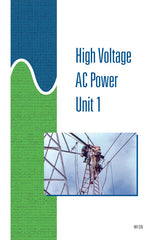Get the Study Guide for this videoFollow the video session-by-session with this handy book. Color illustrations throughout. \$15

Instructor Guide  available

High-Voltage AC Power 1 - Instant Video

Summary

This 58-minute video introduces some of the factors that influence transmission efficiency and power loss. The video explains how T&D systems are designed to minimize power loss and how resistance, capacitive reactance, and inductive reactance can be manipulated to help maintain minimum levels of power loss.

Training Sessions

Factors That Affect Power Loss – 12 minutes
Defines power loss. Explains the relationship between voltage, current, and resistance in an electrical circuit. Explains the relationship of transmission voltage to power loss.

Inductance and Inductive Reactance – 13 minutes
Defines the terms inductance and inductive reactance. Explains how inductance develops in transmission lines. Gives examples of how inductive reactance can be changed in transmission lines.

Capacitance and Capacitive Reactance – 10 minutes
Defines the terms capacitance and capacitive reactance. Explains how capacitance develops in transmission lines. Gives examples of how capacitive reactance can change in transmission lines.

Resistance – 9 minutes
Define resistances. Identifies and explains the factors that determine the resistance of a conductor.

Impedance – 14 minutes
Defines impedance. Describes the relationships between impedance, current, and voltage. Defines resonance. Explains the relationship between impedance and power loss. Defines corona and explains how it can be minimized.

Item Code: HV1-IV